# C++ Program to Find and Print Even Numbers in an Array

## Print even numbers in an array of 10 elements

The question is: create a C++ program that prints even numbers from an array supplied by the user. The answer to this question is the program given below:

```#include<iostream>

using namespace std;
int main()
{
int arr, i;
cout<<"Enter any 10 numbers: ";
for(i=0; i<10; i++)
cin>>arr[i];
cout<<"\nEven Numbers are:\n";
for(i=0; i<10; i++)
{
if(arr[i]%2==0)
cout<<arr[i]<<" ";
}
cout<<endl;
return 0;
}```

The initial output produced by the above C++ program on printing all even numbers available in a given array is shown in the snapshot given below: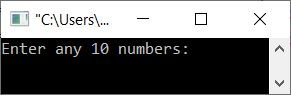Now enter any ten numbers, such as 11, 12, 13, 14, 15, 16, 17, 18, 19, 20, and press the ENTER key to find and print all the even numbers, as shown in the screenshot below: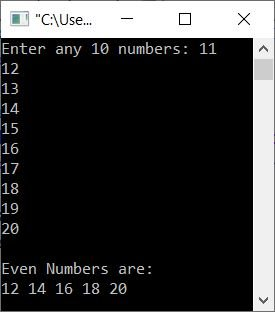The following is the dry run of the above program with the exact same user input as provided above:

• When the user enters 10 elements or numbers, the index-wise storage of these numbers will be arr = 11, arr = 12, arr = 13,... arr = 20.
• Now the execution of the second for loop begins.
• That is, i=0, and the condition i<10 or 0<10 evaluates to be true.
• Therefore, program flow goes inside the loop, and the condition of if gets evaluated.
• That is, because the condition arr[i]%2==0 or arr%2==0 or 11%2==0 or 1==0 evaluates to false, program flow does not proceed to the body of if.
• Now the value of i gets incremented using i++ (third statement of the loop). So i is now equal to 1.
• The condition of the for loop again gets evaluated with a new value of i.
• This time, the condition i<10 or 1<10 evaluates to true as well.
• Therefore, again, program flow goes inside the loop.
• And the condition arr[i]%2==0, arr%2==0, 12%2==0, or 0==0 evaluates to true.
• Therefore, this time, program flow goes inside the if's body.
• And the value of arr[i] or arr, which is 12, gets printed on the output.
• Again, a second time, the value of i gets incremented. Now i=2.
• And the condition i<10 or 2<10 evaluates to true once more.
• Therefore, the program again goes inside the loop.
• This process continues until the condition is evaluated as false.
• In this way, one by one, all even numbers get printed.

## Print even numbers in an array of n numbers

Now let's modify the above program and create a new one that allows the user to define the size of the array. Also, this program prints a message if no even numbers are found.

```#include<iostream>

using namespace std;
int main()
{
int n, i, temp=0;
cout<<"Enter the size of array: ";
cin>>n;
int arr[n];
cout<<"Enter any "<<n<<" numbers: ";
for(i=0; i<n; i++)
cin>>arr[i];
for(i=0; i<n; i++)
{
if(arr[i]%2==0)
{
if(temp==0)
cout<<"\nEven Numbers are:\n";
cout<<arr[i]<<" ";
temp=1;
}
}
if(temp==0)
cout<<endl;
return 0;
}```

A sample run of the above program with user input of size 4 and 1, 3, 5, 7 as four numbers is shown below: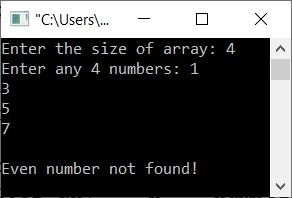Here is another sample run with user input of 6 as size and 11, 22, 33, 44, 55, and 66 as six numbers for the array: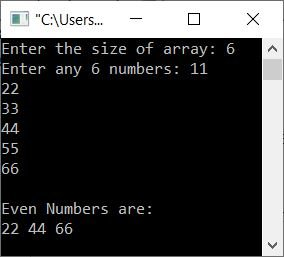## Print even numbers in an array using another array

This program uses another array that stores all the even numbers from the array given by the user. After storing all even numbers from the given array in the second (another) array one by one, Just print the elements of the second array as shown in the program given below:

```#include<iostream>

using namespace std;
int main()
{
int n, i, j=0;
cout<<"Enter the size of array: ";
cin>>n;
int arr[n], eve[n];
cout<<"Enter any "<<n<<" numbers: ";
for(i=0; i<n; i++)
{
cin>>arr[i];
if(arr[i]%2==0)
{
eve[j] = arr[i];
j++;
}
}
if(j==0)
else if(j==1)
cout<<"\nThere is only 1 even number found. That is:\n"<<eve;
else
{
cout<<"\nList of Even Numbers:\n";
for(i=0; i<j; i++)
cout<<eve[i]<<" ";
}
cout<<endl;
return 0;
}```

Here is its sample run with user input of 3 as size and 11, 22, and 33 as three numbers: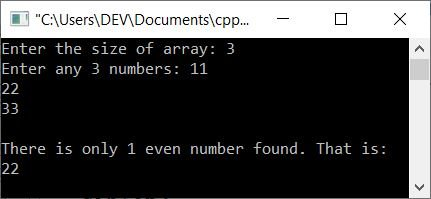C++ Quiz

« Previous Program Next Program »